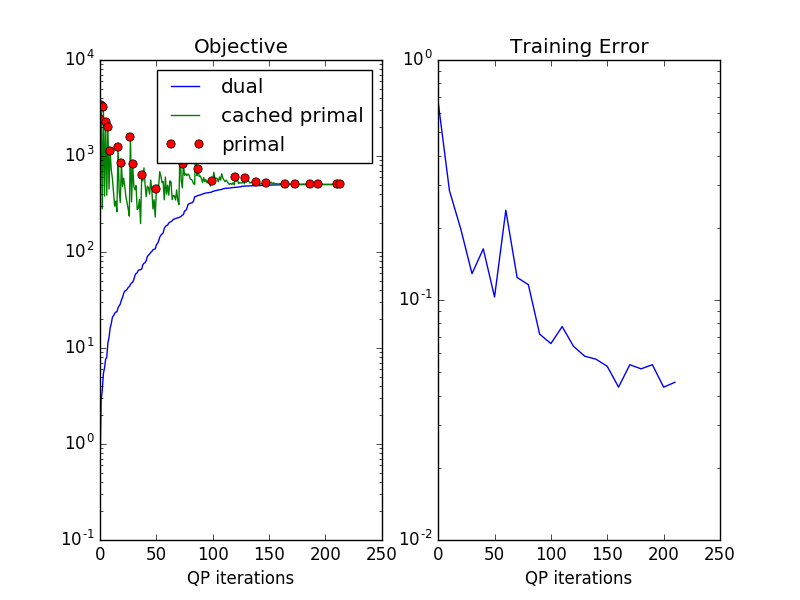# Efficient exact learning of 1-slack SSVMs¶

This example illustrates the role of approximate inference and caching in exact learning of a 1-slack SSVM.

Please see plot_objetive_curve.py for an interpretation of the curves.

We start learning by using an undergenerating inference method, QPBO-based alpha expansion. One the algorithm can not find a violated constraint any more, we switch to a less efficient but exact inference procedure, branch-and-bound based on AD3. The switch to AD3 can be seen in the graph after the (approximate) primal objective and the cutting plane lower bound touch. (zoom in)

After the switch to exact inference, the red circles show the true primal objective.Script output:

```OneSlackSSVM(C=1.0, break_on_bad=False, cache_tol='auto',
check_constraints=False, inactive_threshold=1e-05,
inactive_window=50, inference_cache=100, logger=None,
max_iter=10000,
model=DirectionalGridCRF(n_states: 3, inference_method: ('ad3', {'branch_and_bound': True}), n_features: 3, n_edge_features: 2),
n_jobs=-1, negativity_constraint=None, show_loss_every=10,
Iterations: 214
Objective: 505.245528
Gap: 0.004014
```
```from pystruct.models import DirectionalGridCRF
import pystruct.learners as ssvm
from pystruct.datasets import generate_blocks_multinomial
from pystruct.plot_learning import plot_learning

X, Y = generate_blocks_multinomial(noise=2, n_samples=20, seed=1)
crf = DirectionalGridCRF(inference_method="qpbo", neighborhood=4)
clf = ssvm.OneSlackSSVM(model=crf, n_jobs=-1, inference_cache=100,
show_loss_every=10,
Download Python source code: `plot_exact_learning.py`